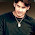# Age Problems - Concepts and Tricks

Age problems are one of the most common topics in IBPS, CAT, GMAT and other banks exams. Students waste lots of time in this question as it look very simple but when they start solving with triditional methods, it takes a lot of time. Today I am solving few solving with shortcut trick. In case of any problem, please comment below.

Best way to solve Age questions is to assume fixed period with which further conditions will be compared. For example taking 2000 as fixed year.

## Application of this rule

### Example 1

Raman's age after 15 years will be 5 times his age 5 years back. What is his present age ?
Solution - Let's assume right now it is year 2000

Age of Raman in 1995 = x
Age of Raman in 2015 = 5x
Present age of Raman (in 2000) = x+5 or 5x-15

we will solve these two equation to find x.

X= 5. Then Raman's present age becomes = x +5 = 10

### Example 2

Rahul was 4 times old as his son 8 years back and he will be 2 times old as his son after 8 years. Calculate Rahul and his son's age.

Assume that currently it is year 2000.
In 1992 Rahul's age = 4x, Age of Rahul's son = x
In 2008 Rahul's age = 2y and Age of Rahul's son = y

Now we get two equations 2y - 4x = 16 and y - x = 16
By solving this equation x = 8, so Rahul' son's current age = 16 years and Rahul's age = 40 years.#### Latest Courses on BankExamsToday1.sir you are just giving two examples for each section please include some more questions thanks in advance :)

1.yes sir plz....

2.How did you get 2x-4y = 16

1.2(x-2y)=16
x-2y=16/2
x-2y=8
x=8 -2y=8
x=8 y=-4

2.buddy u are wrong....can u explain how u got the value...when there are two unknown variables...we cant find the values of both....

3.Use factorisation method

4.you got 2y-4x=16 as
16 means Difference in years 1992 and 2008 ( its 16 yrs ) and
2y= age in 2008
4x= age in 1992
So Take a difference 2y-4x ( For current age ) thats equal to difference in time period i.e, 16 yrs

3.please include some more examples .......

4.Can anybody please answer these questions with explanations. As I am unable to understand and solve these problems.

1. Kalyani got married 6 years ago. Today her age is (1 1/4= 5/4) times her age at the time of marriage. Her son‟s age is (1/10)th times her age.
Her son‟s age is _________
1. 2 yrs 2. 3 yrs 3. 4 yrs 4. 5 yrs

2.Mahesh is as much younger to Anil as he is older to Prashant. If the sum of the ages of Anil and Prashant is 48 years,
what is the age of Mahesh?
1. 20 yrs 2. 24 yrs 3. 30 yrs 4. None

3. Five years ago, Vinay‟s age was one third of the age of Vikas and now Vinay‟s age is 17 years. What is the present
age of Vikas?
1. 5 yrs 2. 36 yrs 3. 41 yrs 4. 51 yrs

4.Siddhika‟s age is 1/6 of her father‟s age. Siddhika‟s father‟s age will be twice of Kamal‟s age after 10 years. IfKamal‟s 8th birthday was celebrated two years before, then what is Siddhika‟s present age?
1. 10 years 2. 15 years 3. 5 years 4. 3 years

5. 3 years ago, the father‟s age was twice the ages of his 4 daughters. In 3 years time, the father‟s age will be equal to
sum of ages of the daughters. Find the present age of father.
1. 45 years 2. 36 years 3. 24 years 4. 39 years

6. Ten years before, one is seven years more than the “half the age” of other. Now the bride‟s age is 30 years. And also
one is nineteenths the age of other. What is groom‟s present age.
1. 35 2. 27 3. 40 4. 58

7. When I add 4 times my age 4 years from now to 5 times my age 5 years from now, I get 10 times my current age.
How old will I be 3 years from now?
1. 52 2. 40 3. 44 4. None

5.1. kalyanis present age is if X than equation comes as X= (X-6)*5/4 so the value of X= 30 like wise age of her son wl b 30*1/10= 3 yrs

6.Hi. Can you please explain, in example 2, from where we get this 16?

7.gud eve sir i`m not understanding the age problem so plzzz can u explain breifle...

8.2.Mahesh is as much younger to Anil as he is older to Prashant. If the sum of the ages of Anil and Prashant is 48 years,
what is the age of Mahesh?
1. 20 yrs 2. 24 yrs 3. 30 yrs 4. None

is ques. ko plz expln krke btaye

1.Mahesh is younger than Anil and Elder than Prashant..
Mahesh's age = x
Prashant's age = x-k
Anil's age = x+k
Anil + Prashant = 48 => x+k+x-k = 48
Mahesh age x=24 years

9.how the x=5 came

Thanks for commenting. Follow us on Telegram. Search BankExamsToday